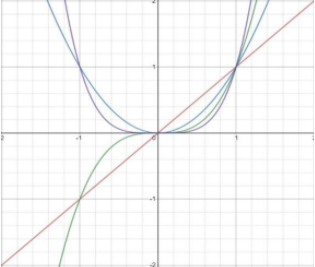QuestionAnswers

# For the real x, let$f(x) = \max \{ x,{x^2},{x^3},{x^4}\}$, then $f(x)$isA. ${x^4}$ for $x \leqslant - 1$B. ${x^2}$ for $- 1 < x \leqslant 0$C. $f(\dfrac{1}{2}) = \dfrac{1}{2}$D. $f(\dfrac{1}{2}) = \dfrac{1}{4}$Verified
130.5k+ views
Hint: The functions which are defined as maximum or minimum of a group of functions can easily be found out by graphical method. This is done by plotting all the functions separately and then applying the condition of maxima or minima as defined in the function.

In such questions first we need to understand the function. Let us understand it in a simpler function which says $f(x) = \max \{ x,{x^2}\}$.
Here, for any value in the domain the higher value among $x$ and ${x^2}$ is the value of the function. For example if we have to find $f(2)$ we get 2 and 4 when put into the function. The higher one among them is 4 so the value of function turns out to be 4.
Similarly, if have to find out $f(\dfrac{1}{3})$ we get $\dfrac{1}{3}$ and $\dfrac{1}{9}$. The higher one among them is $\dfrac{1}{3}$ so the value of function turns out to be $\dfrac{1}{3}$.
Here, we are given a function $f(x) = \max \{ x,{x^2},{x^3},{x^4}\}$. This is a little more complex than the above example function. These types of complex functions can be easily solved by graphical methods.
For graphical methods first we need to make a graph of every sub-function and then find the maximum of it.Purple line = ${x^4}$
Green line = ${x^3}$
Blue line = ${x^2}$
Red line = ${x^{}}$
Now, from the graph it can be seen that in $x \leqslant - 1$ ,${x^4}$ is maximum. Therefore option (a) is correct.
Similarly, from the graph it can be seen that in $- 1 < x \leqslant 0$ , ${x^2}$is maximum. Therefore option (b) is correct.
Now, the graph shows that in the domain $0 < x \leqslant 1$ the function is maximum in the red line which follows the function $f(x) = x$. Therefore, $f(\dfrac{1}{2}) = \dfrac{1}{2}$ and option (c) is correct and (d) incorrect.

Hence, option (a), (b) and (c) are correct.

Note:The functions involving maxima or minima of sub-functions can be solved easily by graphical methods. While choosing maximum or minimum from the graph extra attention should be taken.